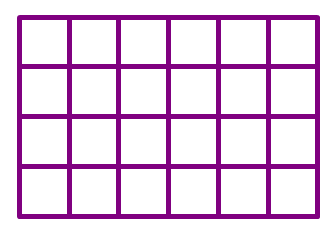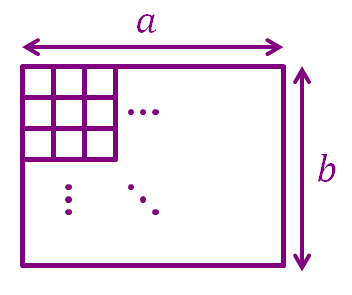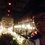# How to Count Squares!

Let me go grab a hamburger real quick...

Ok, I'm back.

How many squares are there in the $6\times4$ grid below?That's a reaallly good question!

Let's start by counting the smallest $1\times1$ squares, this is just the same as counting the number of unit squares in a $6\times4$ grid, there are $6\times4=24$ 1 by 1 squares in the grid.

Now let's move on to the $2\times2$ squares, notice that counting the number of $2\times2$ squares in a $6\times4$ grid is just the same as counting the number of unit squares in a $5\times3$ grid. So the number of 2 by 2 squares in the grid is $5\times3=15$.

Now the $3\times3$ squares, similarly, counting the number of $3\times3$ squares in a $6\times4$ grid is just the same as counting the number of unit squares in a $4\times2$ grid, which is $4\times2=8$.

And again the number of $4\times4$ squares in a $6\times4$ grid is equal to the number of unit squares in a $3\times1$ grid, which is $3\times1=3$.

Add up all the number of squares together: $24+15+8+3=50$. Tada! We now have our answer! There are 50 squares in a $6\times4$ grid.

Mmm... the hamburger is really good...

Back on topic, in general, what is the total number of squares in an $a\times b$ grid (where $a$ is the width of the grid and $b$ is the height of the grid), given $a\geqslant b$?Again let's start from the $1\times1$ squares, that's trivial, there's $ab$ of them.

Now moving on to the $2\times2$ squares, the number of $2\times2$ squares in an $a\times b$ grid is equal to the number of unit squares in an $(a-1)\times(b-1)$ grid.

Notice the pattern? Counting the number of $n\times n$ squares in an $a\times b$ grid is the same as counting the number of unit squares in an $(a-n+1)\times(b-n+1)$ grid.

The largest square that can contain in an $a\times b$ grid given that $a\geqslant b$ is $b\times b$.

Hence, the total number of squares in an $a\times b$ grid is $ab+(a-1)(b-1)+(a-2)(b-2)+\ldots+[a-(b-2)][b-(b-2)]+[a-(b-1)][b-(b-1)]$ Or $\sum_{i=0}^{b-1}{(a-i)(b-i)}$

This is ugly, we don't like sigma symbols sitting around, so why not we simplify this a little bit...

\begin{aligned} \sum_{i=0}^{b-1}{(a-i)(b-i)}&=\sum_{i=0}^{b-1}{[ab-(a+b)i+i^2]} \\&=ab^2-\frac{(a+b)b(b-1)}{2}+\frac{b(b-1)(2b-1)}{6} \\&=b\left[ab-\frac{ab-a+b^2-b}{2}+\frac{2b^2-3b+1}{6}\right] \\&=\frac{b}{6}\left[6ab-3ab+3a-3b^2+3b+2b^2-3b+1\right] \\&=\frac{b}{6}\left[3ab+3a-b^2+1\right] \\&=\frac{b(b+1)(3a-b+1)}{6} \end{aligned} BOOM! There we have it! *Round of applause* *Fireworks* *Pancakes*

The total number of squares in an $a\times b$ grid (where $a$ is the width of the grid and $b$ is the height of the grid), given $a\geqslant b$ is $\frac{b(b+1)(3a-b+1)}{6}$

If $a, then we just swap $a$ and $b$.

Special case: If $a=b$, the above equation becomes $\frac{a(a+1)(2a+1)}{6}$ which is the formula for the sum of squares from 1 to $a$.

Done! Now let me finish my burger...

##### This is one part of Quadrilatorics.Note by Kenneth Tan
5 years, 3 months ago

This discussion board is a place to discuss our Daily Challenges and the math and science related to those challenges. Explanations are more than just a solution — they should explain the steps and thinking strategies that you used to obtain the solution. Comments should further the discussion of math and science.

When posting on Brilliant:

• Use the emojis to react to an explanation, whether you're congratulating a job well done , or just really confused .
• Ask specific questions about the challenge or the steps in somebody's explanation. Well-posed questions can add a lot to the discussion, but posting "I don't understand!" doesn't help anyone.
• Try to contribute something new to the discussion, whether it is an extension, generalization or other idea related to the challenge.
• Stay on topic — we're all here to learn more about math and science, not to hear about your favorite get-rich-quick scheme or current world events.

MarkdownAppears as
*italics* or _italics_ italics
**bold** or __bold__ bold
- bulleted- list
• bulleted
• list
1. numbered2. list
1. numbered
2. list
Note: you must add a full line of space before and after lists for them to show up correctly
paragraph 1paragraph 2

paragraph 1

paragraph 2

[example link](https://brilliant.org)example link
> This is a quote
This is a quote
    # I indented these lines
# 4 spaces, and now they show
# up as a code block.

print "hello world"
# I indented these lines
# 4 spaces, and now they show
# up as a code block.

print "hello world"
MathAppears as
Remember to wrap math in $$ ... $$ or $ ... $ to ensure proper formatting.
2 \times 3 $2 \times 3$
2^{34} $2^{34}$
a_{i-1} $a_{i-1}$
\frac{2}{3} $\frac{2}{3}$
\sqrt{2} $\sqrt{2}$
\sum_{i=1}^3 $\sum_{i=1}^3$
\sin \theta $\sin \theta$
\boxed{123} $\boxed{123}$

## Comments

Sort by:

Top Newest

You should add this to the Brilliant wiki. Great note!

- 5 years, 3 months ago

Log in to reply

Cool! Thanks for that note bro, you're awesome!

- 5 years, 3 months ago

Log in to reply

Hope you finished your burger peacefully :P

- 5 years, 3 months ago

Log in to reply

Thanks, I'm glad you liked the note. Well unfortunately, I think my hamburger has become stale. XD

- 5 years, 3 months ago

Log in to reply

جميلة

- 5 years, 3 months ago

Log in to reply

Translation: beautiful!

- 5 years, 3 months ago

Log in to reply

HEY tankenneth, you hyped?

- 5 years, 3 months ago

Log in to reply

Oh yes I am! $1+1=3$

- 5 years, 3 months ago

Log in to reply

are you a robot, cos I need some real friends? Humanity is a lie, the computer generation is upon us. Support the cause m64^(1/2)

- 5 years, 3 months ago

Log in to reply

No, i am 100.1% sure I'm not a robot.

- 5 years, 3 months ago

Log in to reply

What a note @Tan Kenneth:)

- 5 years, 3 months ago

Log in to reply

Nice simple way of explaining complex situation. So many thanks.

- 5 years, 1 month ago

Log in to reply

×

Problem Loading...

Note Loading...

Set Loading...